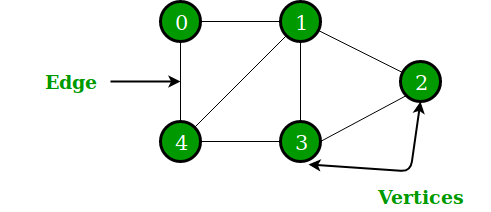Open In App

# Applications of Graph Data Structure

A graph is a non-linear data structure, which consists of vertices(or nodes) connected by edges(or arcs) where edges may be directed or undirected.• In Computer science graphs are used to represent the flow of computation.
• Google maps uses graphs for building transportation systems, where intersection of two(or more) roads are considered to be a vertex and the road connecting two vertices is considered to be an edge, thus their navigation system is based on the algorithm to calculate the shortest path between two vertices.
• In Facebook, users are considered to be the vertices and if they are friends then there is an edge running between them. Facebook’s Friend suggestion algorithm uses graph theory. Facebook is an example of undirected graph.
• In World Wide Web, web pages are considered to be the vertices. There is an edge from a page u to other page v if there is a link of page v on page u. This is an example of Directed graph. It was the basic idea behind Google Page Ranking Algorithm.
• In Operating System, we come across the Resource Allocation Graph where each process and resources are considered to be vertices. Edges are drawn from resources to the allocated process, or from requesting process to the requested resource. If this leads to any formation of a cycle then a deadlock will occur.
• In mapping system we use graph. It is useful to find out which is an excellent place from the location as well as your nearby location. In GPS we also use graphs.
• Facebook uses graphs. Using graphs suggests mutual friends. it shows a list of the f following pages, friends, and contact list.
• Microsoft Excel uses DAG means Directed Acyclic Graphs.
• In the Dijkstra algorithm, we use a graph. we find the smallest path between two or many nodes.
• On social media sites, we use graphs to track the data of the users. liked showing preferred post suggestions, recommendations, etc.
• Graphs are used in biochemical applications such as structuring of protein, DNA etc.

Thus the development of algorithms to handle graphs is of major interest in the field of computer science.

Three Applications of Graphs in the area of computer engineering:

1. The applications of graph split broadly into three categories:

a) First, analysis to determine structural properties of a network, such as the distribution of vertex degrees and the diameter of the graph. A vast number of graph measures exist.

b) Second, analysis to find a measurable quantity within the network, for example, for a transportation network, the level of vehicular flow within any portion of it.

c) Third, analysis of dynamic properties of network. Map of a country can be represented using a graph. Road network, Air network or rail network can be represented using a graph. Connection among routers in a communication network can be represented using a graph. Routing of a packet between two communicating nodes can be done through the shortest path.

2. Graph theory is useful in biology and conservation efforts where a vertex can represent regions where certain species exist and the edges represent migration paths, or movement between the regions. This information is important when looking at breeding patterns or tracking the spread of disease.

3. Different activities of a project can be represented using a graph. This graph can be useful in project scheduling.